Examples

Chapter 14 Class 11 Probability
Serial order wise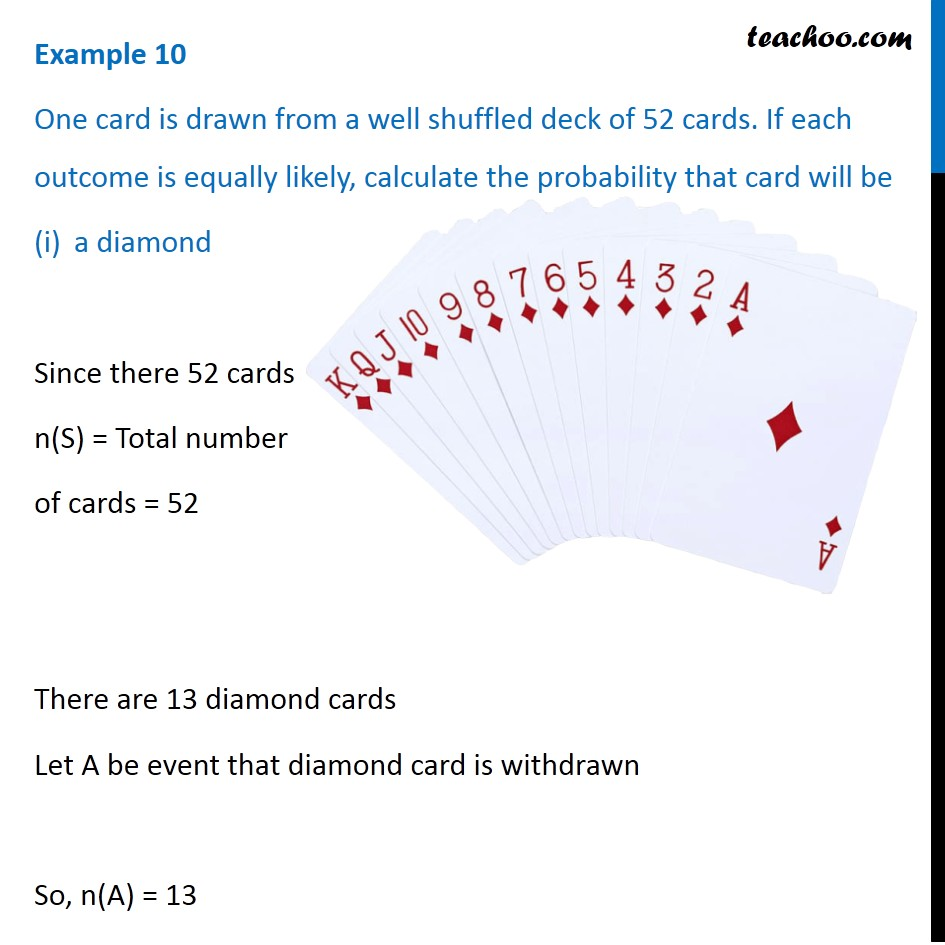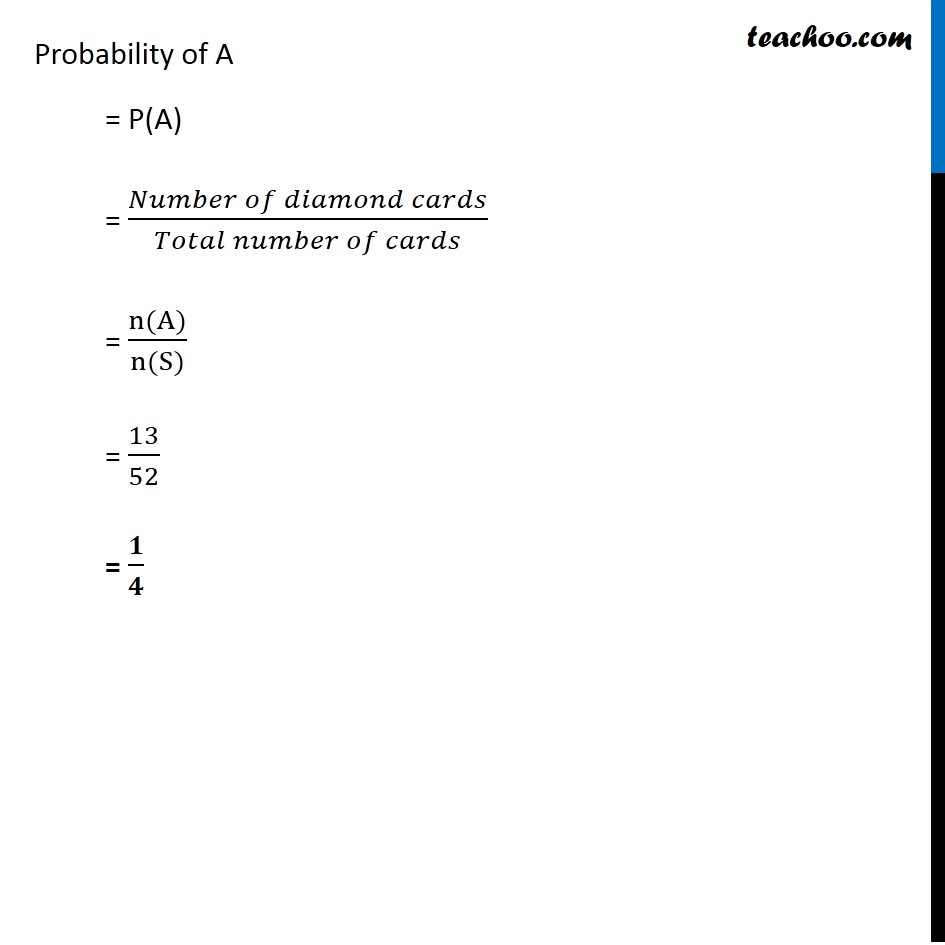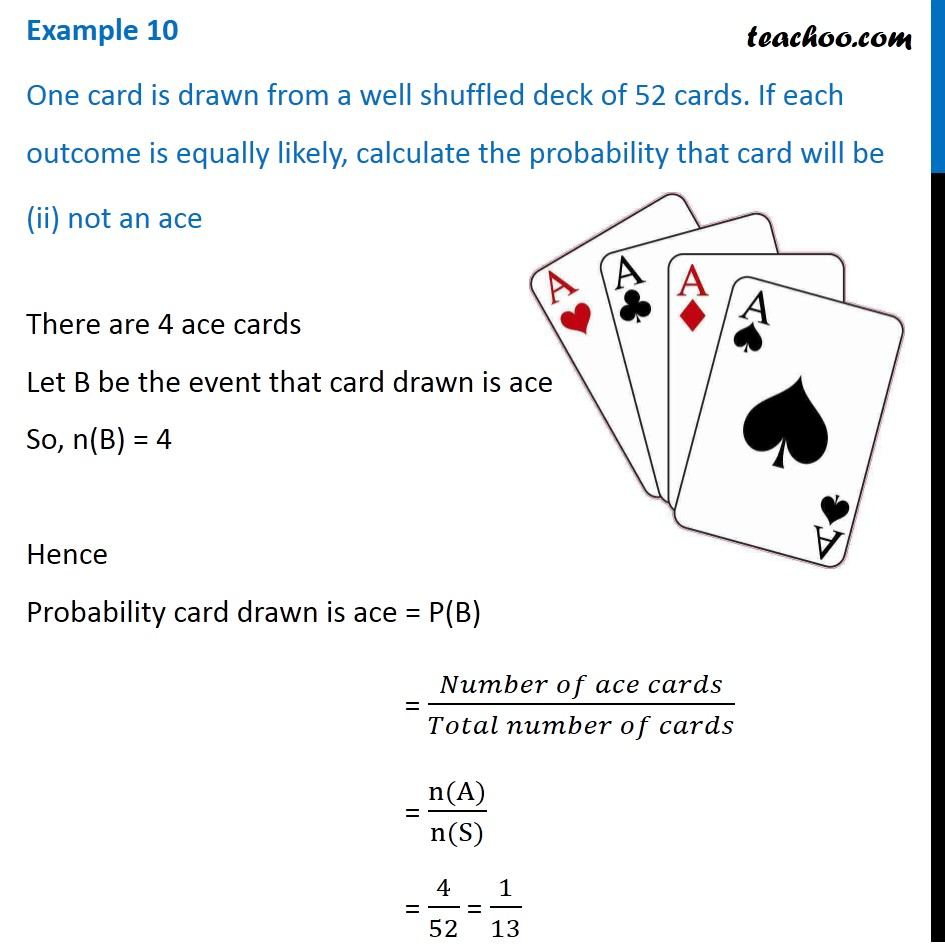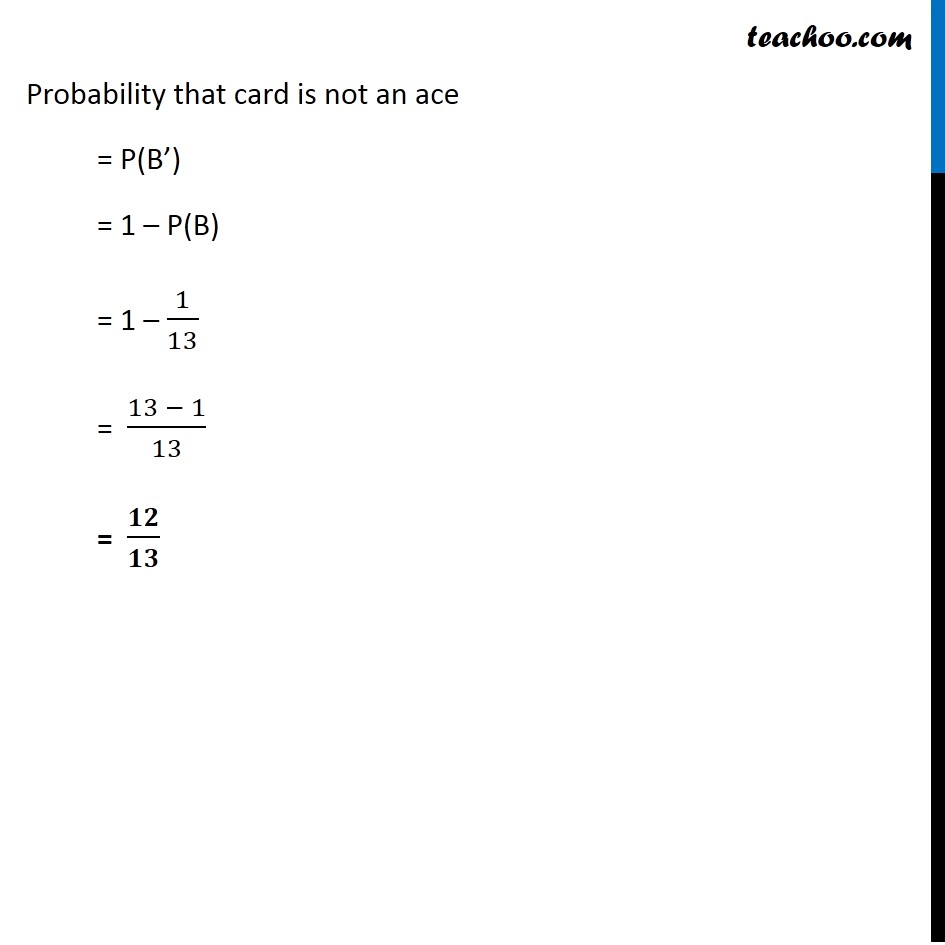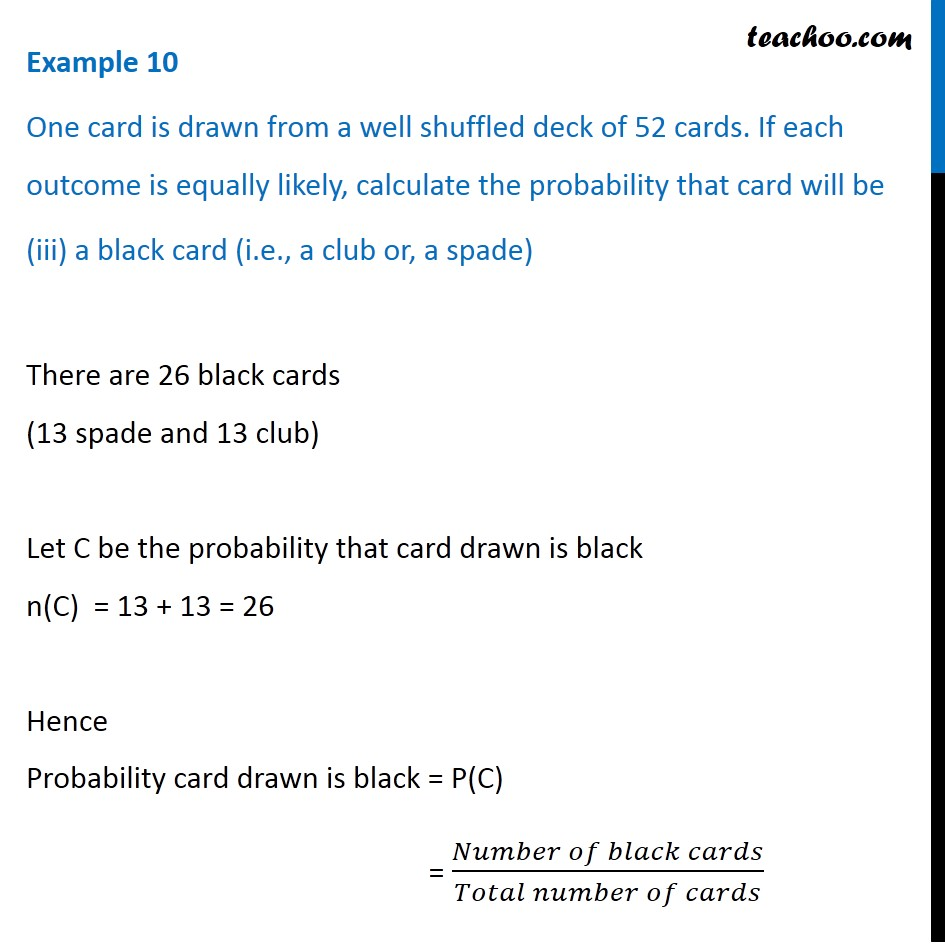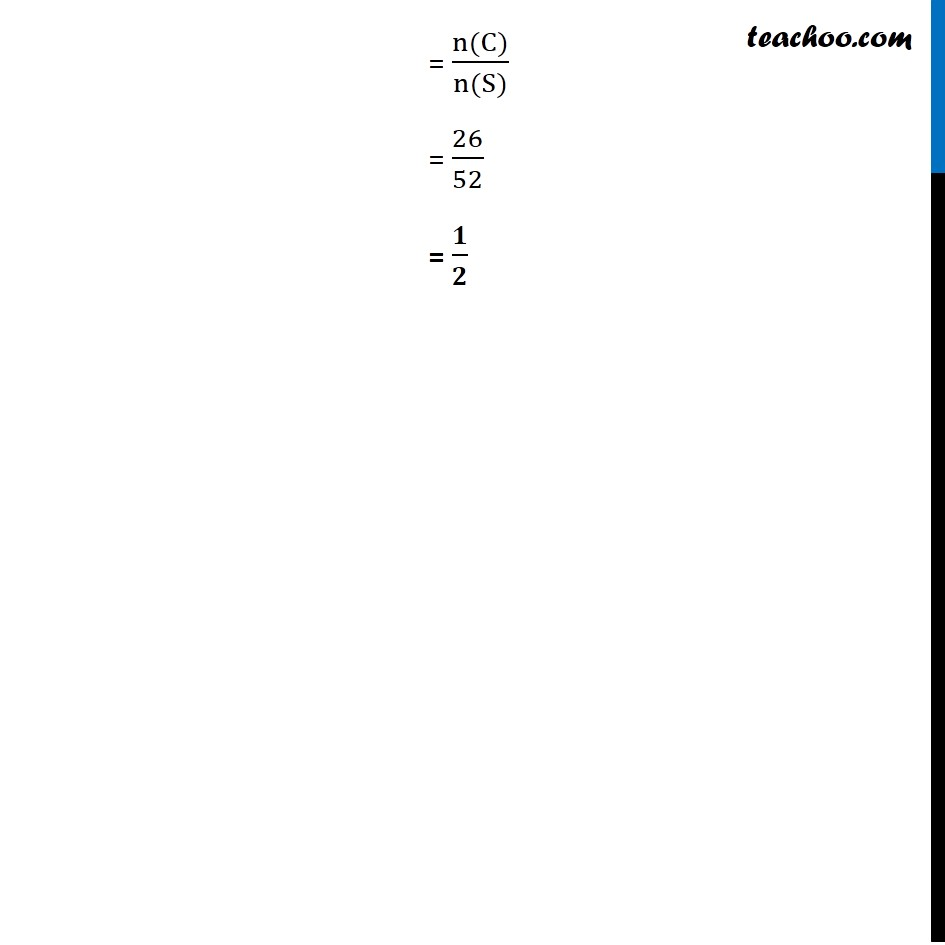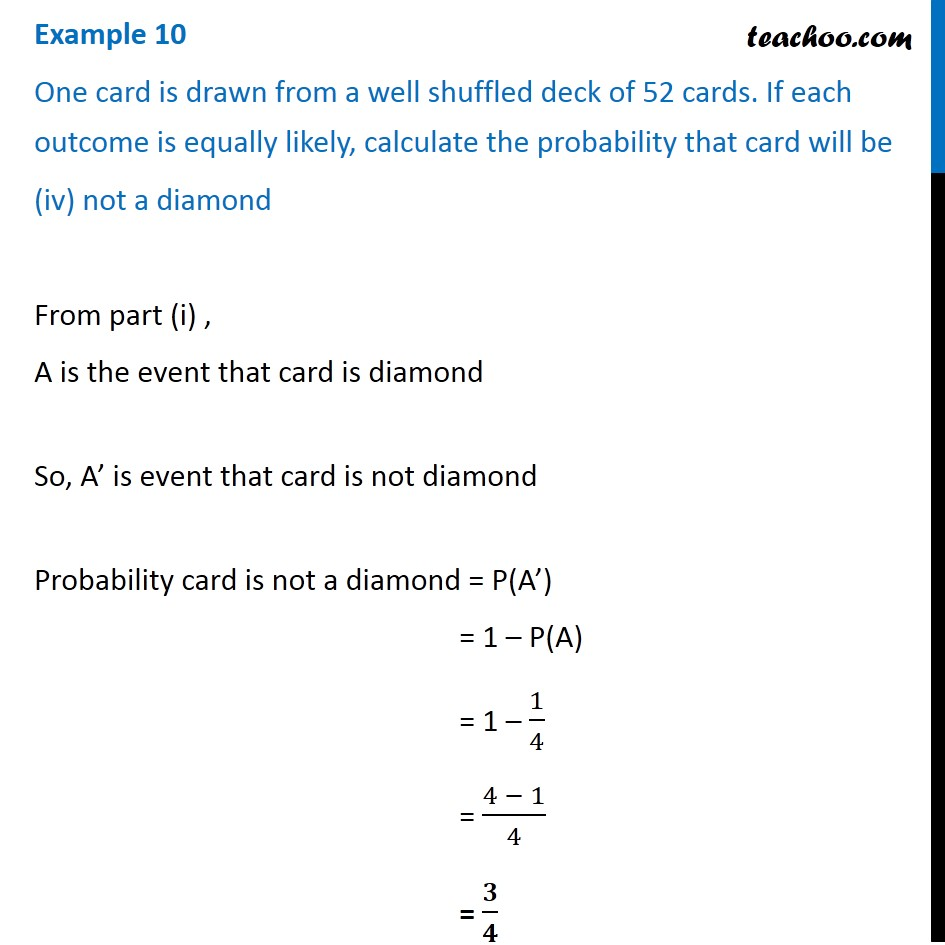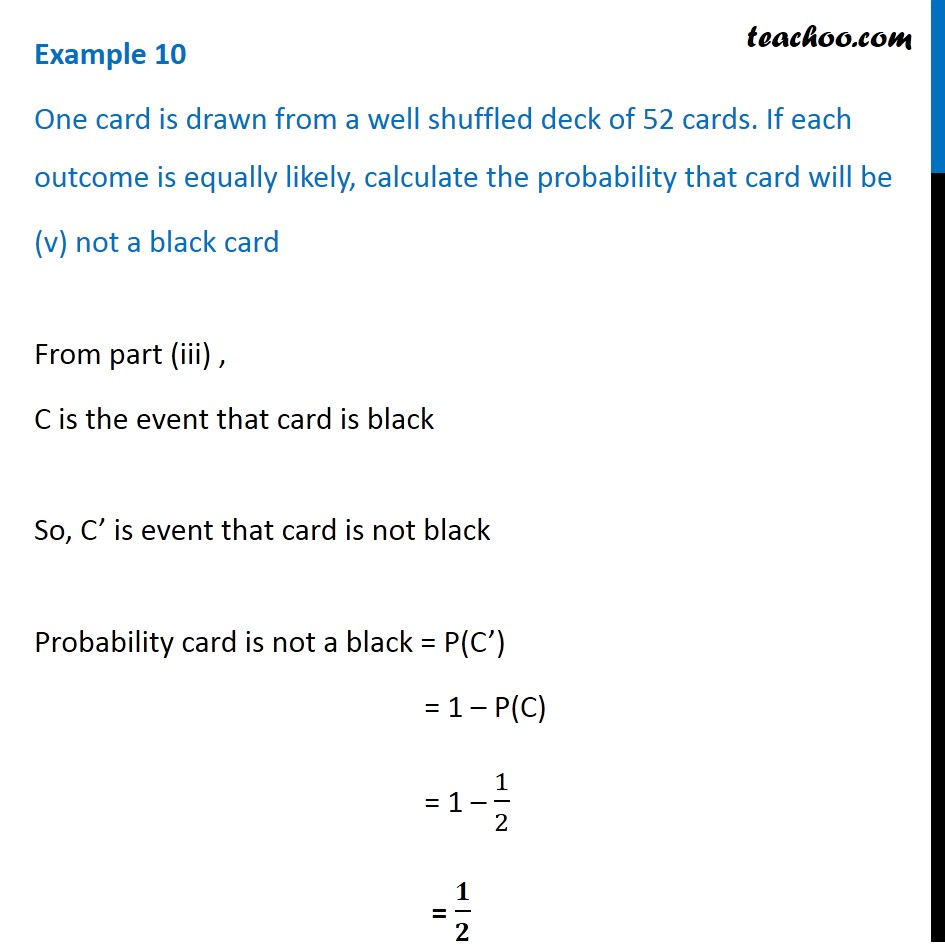Learn in your speed, with individual attention - Teachoo Maths 1-on-1 Class

### Transcript

Example 5 One card is drawn from a well shuffled deck of 52 cards. If each outcome is equally likely, calculate the probability that card will be a diamond Since there 52 cards n(S) = Total number of cards = 52 There are 13 diamond cards Let A be event that diamond card is withdrawn So, n(A) = 13 Probability of A = P(A) = (𝑁𝑢𝑚𝑏𝑒𝑟 𝑜𝑓 𝑑𝑖𝑎𝑚𝑜𝑛𝑑 𝑐𝑎𝑟𝑑𝑠)/(𝑇𝑜𝑡𝑎𝑙 𝑛𝑢𝑚𝑏𝑒𝑟 𝑜𝑓 𝑐𝑎𝑟𝑑𝑠) = (n(A))/(n(S)) = 13/52 = 𝟏/𝟒 Example 5 One card is drawn from a well shuffled deck of 52 cards. If each outcome is equally likely, calculate the probability that card will be (ii) not an ace There are 4 ace cards Let B be the event that card drawn is ace So, n(B) = 4 Hence Probability card drawn is ace = P(B) = (𝑁𝑢𝑚𝑏𝑒𝑟 𝑜𝑓 𝑎𝑐𝑒 𝑐𝑎𝑟𝑑𝑠)/(𝑇𝑜𝑡𝑎𝑙 𝑛𝑢𝑚𝑏𝑒𝑟 𝑜𝑓 𝑐𝑎𝑟𝑑𝑠) = (n(A))/(n(S)) = 4/52 = 1/13 Probability that card is not an ace = P(B’) = 1 – P(B) = 1 – 1/13 = (13 − 1)/13 = 𝟏𝟐/𝟏𝟑 Example 5 One card is drawn from a well shuffled deck of 52 cards. If each outcome is equally likely, calculate the probability that card will be (iii) a black card (i.e., a club or, a spade) There are 26 black cards (13 spade and 13 club) Let C be the probability that card drawn is black n(C) = 13 + 13 = 26 Hence Probability card drawn is black = P(C) = (𝑁𝑢𝑚𝑏𝑒𝑟 𝑜𝑓 𝑏𝑙𝑎𝑐𝑘 𝑐𝑎𝑟𝑑𝑠)/(𝑇𝑜𝑡𝑎𝑙 𝑛𝑢𝑚𝑏𝑒𝑟 𝑜𝑓 𝑐𝑎𝑟𝑑𝑠) = (n(C))/(n(S)) = 26/52 = 𝟏/𝟐 Example 5 One card is drawn from a well shuffled deck of 52 cards. If each outcome is equally likely, calculate the probability that card will be (iv) not a diamond From part (i) , A is the event that card is diamond So, A’ is event that card is not diamond Probability card is not a diamond = P(A’) = 1 – P(A) = 1 – 1/4 = (4 − 1)/4 = 𝟑/𝟒 Example 5 One card is drawn from a well shuffled deck of 52 cards. If each outcome is equally likely, calculate the probability that card will be (v) not a black card From part (iii) , C is the event that card is black So, C’ is event that card is not black Probability card is not a black = P(C’) = 1 – P(C) = 1 – 1/2 = 𝟏/𝟐